# Linearization of the product of two variables

Often when writing a model, the most straightforward way of writing a constraint is by multiplying two variables. Then, in order to solve the model we need to linearize it. There comes the problem, as I always have problems reminding how to linearize a product of variables.

# Linearizing the product of two binary variables

Suppose your model has the product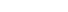, where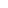,and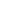are binary. There is an easy way of linearizing that equation. Add the three inequalities below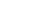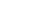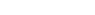The first two inequalities ensure thatwill be zero if eitherorare zero. The last inequality will make sure thatwill take value 1 if both binary variables are set to 1.

# Linearizing the product of a binary and a continuous variable

Now suppose your expression is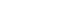, where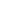is a continuous variable andis your binary variable.

Ifis bounded below by zero and above by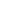(or any Big M), than it is pretty simple: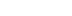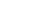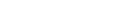Note that ifis zero, than the first inequality ensures thatwill be zero as well (note that the third inequality only states thathas to be greater than a negative number). On the other hand, ifis 1, the first inequality ensures thatis less than our Big M, which is further tightened by our second inequality. The last inequality states then thathas to be greater than or equal to, exactly as we wanted.

Finally, ifis not bounded below by 0, but has bounds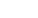(withassumed to be positive due to the last inequality), then the form of the linearized inequalities are the following: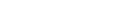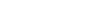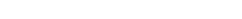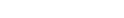If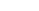thanhas to be zero as well. See how this is automatically enforced by the second inequality. Now if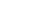thanhas to be equal to. See how this is also enforced by the third inequality. The others are there to ensure everything work and I leave their interpretation for you.

Enjoy it!

(thanks, Arindam!)

This was useful? Buy me a cup of coffee to help me keep this website running!